##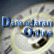Debt Ratio Regression: January 2022

Variables used in the regression

1. Debt Ratio = Debt/ (Market Value of Equity + Debt): If you can get market value of debt, use it. Else, use book value of debt.
2. Payout Ratio= Dividends/ Net Income, if Net Income is positve, not available if net income is negative.
3. Expected growth rate in EPS- next 5 years= You can use expected or even historical earnings growth, if you don't have an EPS growth forecast
4. Effective Tax Rate = Effective tax rate in most recent year

US Regression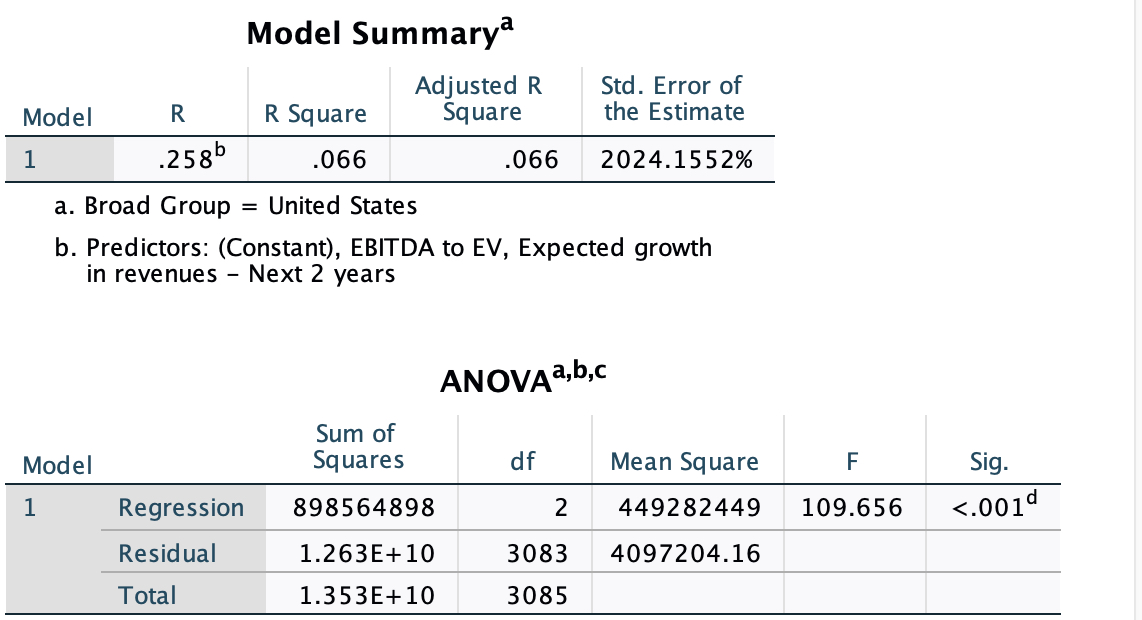## US Regression

####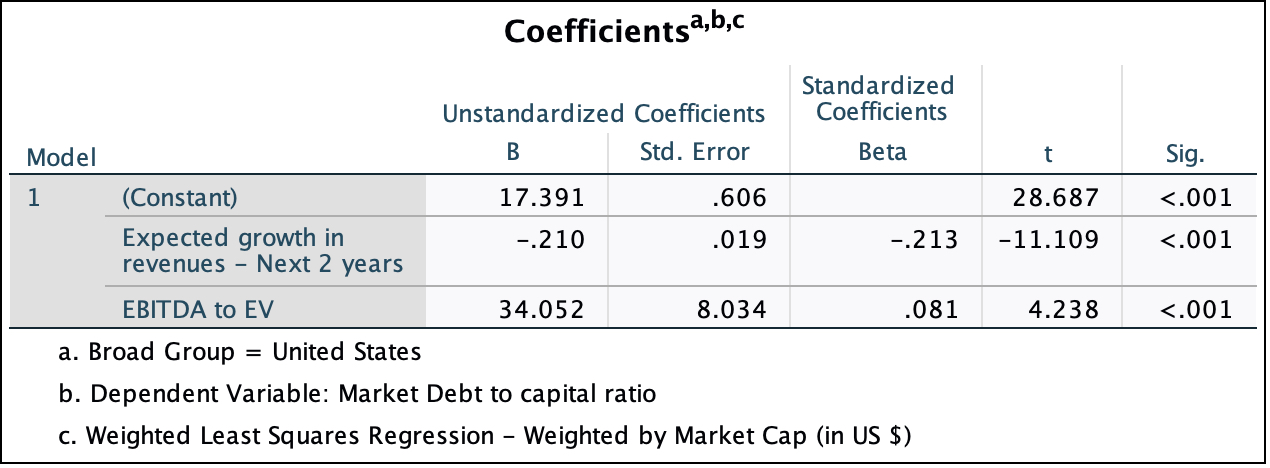Global Regression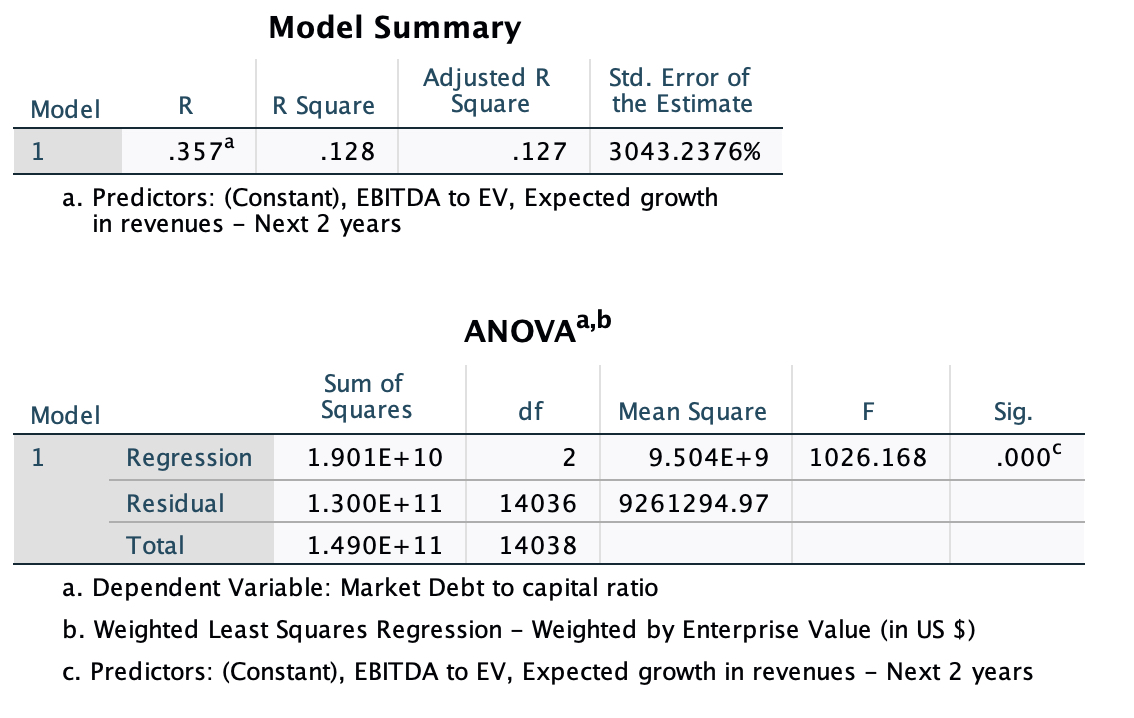## Global Regression

####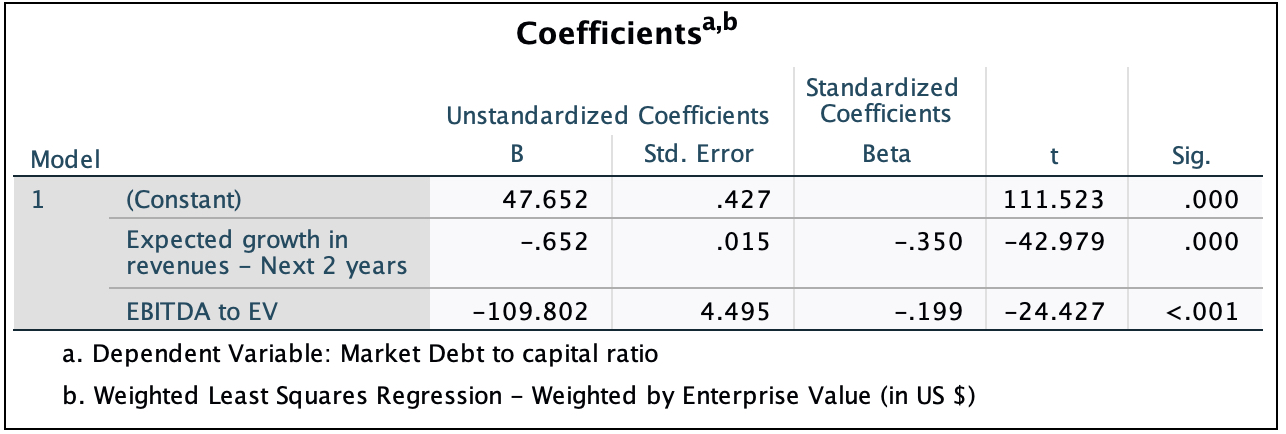• How do I use this regression?

Assume that you want to estimate the market debt ratio for a firm with the following characteristics, using the Global regression

EBITDA/EV = 0.15

Expected growth rate in EPS = 12%

• Predicted Value

Expected Debt Ratio =  47.65 - 0.65 ( 12) -109.80 (.15) =23.38 or 23.38%

If your predicted value is less than zero, your predicted debt ratio is zero.